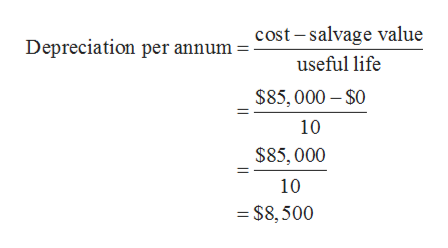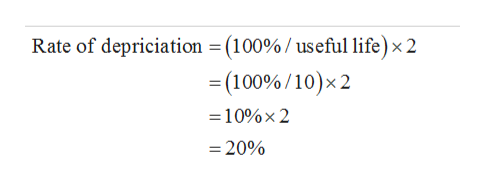# Can someone help me pleaseA Kubota tractor acquired on January 8 at a cost of \$85,000 has an estimated useful life of 10 years. Assuming that it will have no residual value.a.  Determine the depreciation for each of the first two years by the straight-line method.First YearSecond Year\$\$b.  Determine the depreciation for each of the first two years by the double-declining-balance method.First YearSecond Year\$\$

Question
4 views

A Kubota tractor acquired on January 8 at a cost of \$85,000 has an estimated useful life of 10 years. Assuming that it will have no residual value.

a.  Determine the depreciation for each of the first two years by the straight-line method.

 First Year Second Year \$ \$

b.  Determine the depreciation for each of the first two years by the double-declining-balance method.

 First Year Second Year \$ \$
check_circle

Step 1

Depreciation refers to the amount of permanent loss in the value of an asset. It is reduction in the value of an asset which can be due to natural calamity, wear n tear, handling issues.

Under straight-line depreciation method, the rate of depreciation remains same in each year.

Under double-declining-balance method, the depreciation is charged on the book value at double the rate of depreciation calculated under straight-line method.

Step 2

Cost of tractor: \$85,000

Useful life: 10 years

Salvage value: \$0

a)

Depreciation per annum is calculated as follows:help_outlineImage Transcriptionclosecost - salvage value Depreciation per annum = useful life \$85, 000 – \$0 10 \$85,000 10 = \$8,500 fullscreen
Step 3

Depreciation under straight-line method remains same every year. Therefore, depreciation for two years will be \$8,500 ...help_outlineImage TranscriptioncloseRate of depriciation = (100% / useful life)x 2 = (100% /10)×2 =10%× 2 = 20% fullscreen

### Want to see the full answer?

See Solution

#### Want to see this answer and more?

Solutions are written by subject experts who are available 24/7. Questions are typically answered within 1 hour.*

See Solution
*Response times may vary by subject and question.
Tagged in